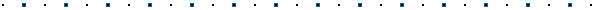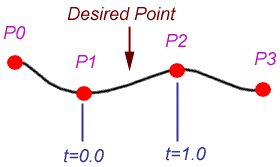#Written by Robert Dunlop Microsoft DirectX MVP## Introduction

Splines are a mathematical means of representing a curve, by specifying a series of points at intervals along the curve and defining a function that allows additional points within an interval to be calculated.  There are various functions available to approximate a curve, but in this article we will focus on a variety of spline known as the Catmull-Rom spline.

## Catmull-Rom Splines

The points that define a spline are known as "Control Points".  One of the features of the Catmull-Rom spline is that the specified curve will pass through all of the control points - this is not true of all types of splines.Figure 1 To calculate a point on the curve, two points on either side of the desired point are required, as shown on the left.  The point is specified by a value t that signifies the portion of the distance between the two nearest control points.

Given the control points P0, P1, P2, and P3, and the value t, the location of the point can be calculated as (assuming uniform spacing of control points):

 q(t) = 0.5 * (1.0f,t,t2,t3)  * [  0 2 0 0 ] [P0] [ -1 0 1 0 ] * [P1] [  2 -5 4 -1 ] [P2] [ -1 3 -3 1 ] [P3] Equation 1

To put that another way:

 q(t) = 0.5 *( (2 * P1) + (-P0 + P2) * t + (2*P0 - 5*P1 + 4*P2 - P3) * t2 + (-P0 + 3*P1- 3*P2 + P3) * t3) Equation 2

This formula gives Catmull-Rom spline the following characteristics:The spline passes through all of the control points. (see Fig. 1)The spline is C1 continuous, meaning that there are no discontinuities in the tangent direction and magnitude. (See Fig. 2)The spline is not C2 continuous.  The second derivative is linearly interpolated within each segment, causing the curvature to vary linearly over the length of the segment.Points on a segment may lie outside of the domain of P1 -> P2. (See Fig. 2)Figure 2

While a spline segment is defined using four control points, a spline may have any number of additional control points.  This results in a continuous chain of segments, each defined by the two control points that form the endpoints of the segments, plus an additional control point on either side of the endpoints.  Thus for a given segment with endpoints Pn and Pn+1, the segment would be calculated using [Pn-1, Pn, Pn+1, Pn+2].

Because a segment requires control points to the outside of the segment endpoints, the segments at the extreme ends of the spline cannot be calculated.  Thus, for a spline with control points 1 through N, the minimum segment that can be formulated is P1<->P2, and the maximum segment is PN-3<->PN-2.   Thus, to define S segments, S+3 control points are required.

## Support of Catmull-Rom Splines in DirectX

The D3DX math libraries provide functions for calculating a point on a Catmull-Rom segment, using 2D, 3D, or 4D vectors.  These functions are:

 D3DXVec2CatmullRom(D3DXVECTOR2* pOut,                    CONST D3DXVECTOR2* pV1,                    CONST D3DXVECTOR2* pV2,                    CONST D3DXVECTOR2* pV3,                    CONST D3DXVECTOR2* pV4,                    FLOAT s)D3DXVec3CatmullRom(D3DXVECTOR3* pOut,                    CONST D3DXVECTOR3* pV1,                    CONST D3DXVECTOR3* pV2,                    CONST D3DXVECTOR3* pV3,                    CONST D3DXVECTOR3* pV4,                    FLOAT s) D3DXVec4CatmullRom(D3DXVECTOR4* pOut,                    CONST D3DXVECTOR4* pV1,                    CONST D3DXVECTOR4* pV2,                    CONST D3DXVECTOR4* pV3,                    CONST D3DXVECTOR4* pV4,                    FLOAT s)

Each of these functions accepts four control points and a positional weight from 0.0 to 1.0, and returns an interpolated vector .  The returned value will fall in the range of *pV2 (when s==0.0) to *pV3 (when s==1.0).

## Sample: Path Interpolation using Catmull-Rom Splines

 The downloadable demo available below demonstrates the use of a Catmull-Rom spline for path interpolation of a vehicle traveling down an infinite highway.  Use the up/down arrow keys to control rate of travel.  You can toggle between Catmull-Rom based interpolation and linear interpolation using the buttons on the right side, to see the difference that spline interpolation makes in providing a smooth animation path.Source code, pre-compiled executable and media included.Download Demo lightmap.zip 347KBRequires DirectX 8.0 Runtimes or LaterThis site, created by DirectX MVP Robert Dunlop and aided by the work of other volunteers, provides a free on-line resource for DirectX programmers.

Special thanks to WWW.MVPS.ORG, for providing a permanent home for this site.

#####Visitors Since 1/1/2000:Last updated: 07/26/05.